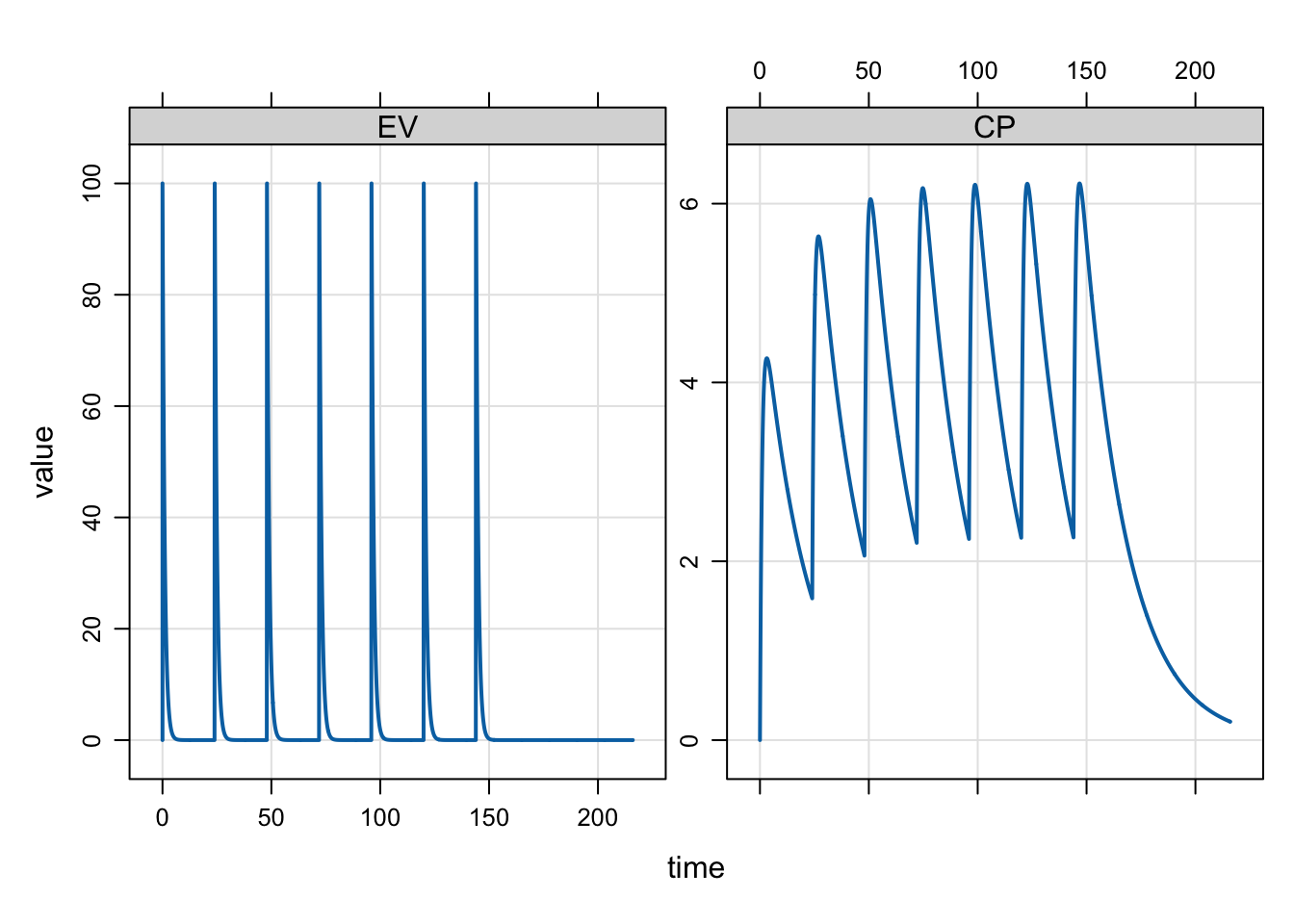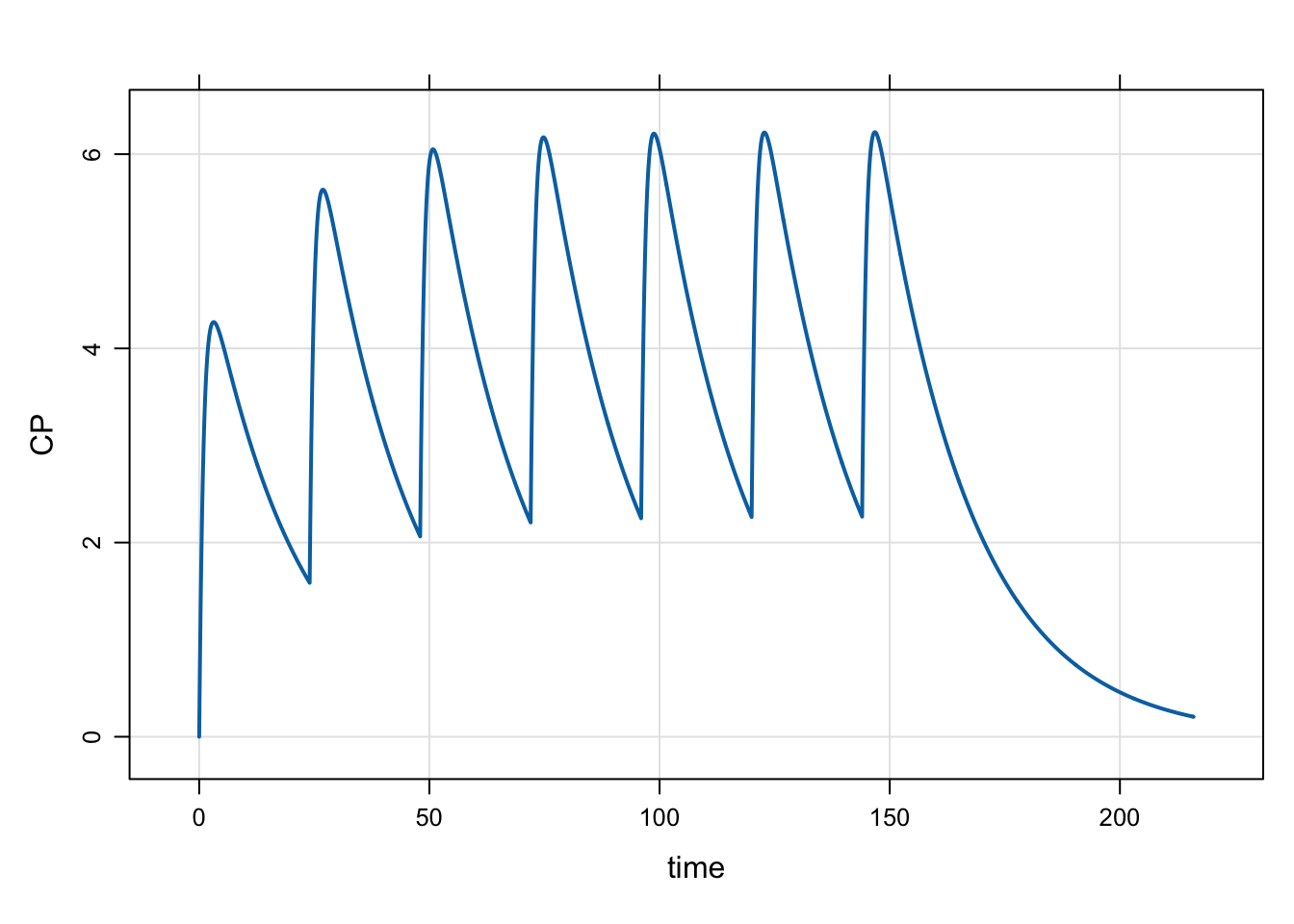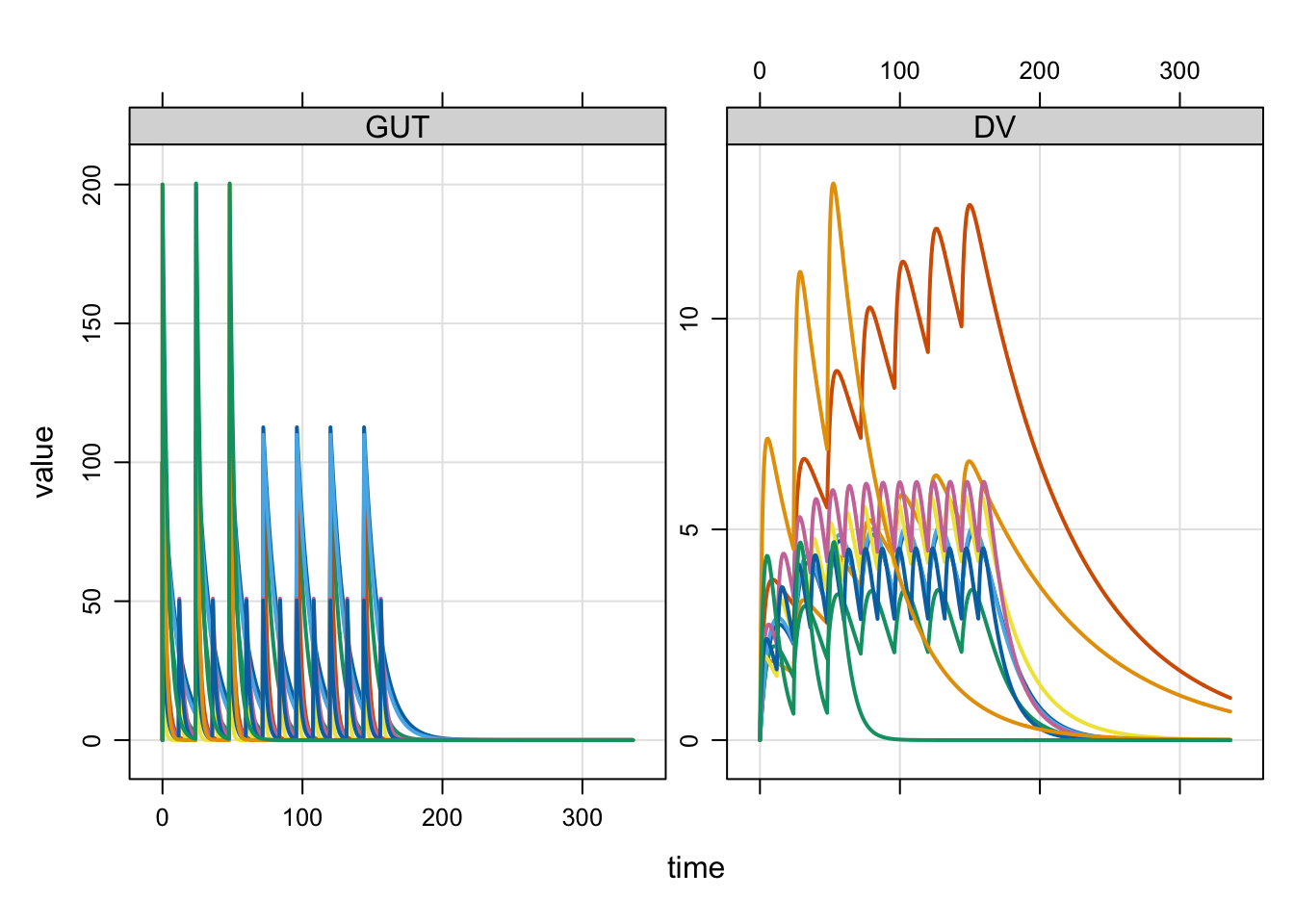# Work with event objects

event objects are data.frame-like data structures that allow you to quickly and flexibly include dosing interventions into your simulations.

events
Author

Kyle Baron

Published

January 1, 2016

``````library(mrgsolve)
library(dplyr)
options(mrgsolve.soloc="build")``````

# 1 Introduction

Event objects are simple ways to implement PK dosing events into your model simulation.

# 2 Setup

Let’s illustrate event objects with a one-compartment, PK model. We read this model from the `mrgsolve` internal model library.

``mod <- mread_cache("pk1cmt", modlib(), end=216, delta=0.1)``

# 3 Events

Events are constructed with the `ev` function

``e <- ev(amt=100, ii=24, addl=6)``

This will implement 100 unit doses every 24 hours for a total of 7 doses.
`e` has class `ev`, but really it is just a data frame

``e``
``````Events:
time amt ii addl cmt evid
1    0 100 24    6   1    1``````
``as.data.frame(e)``
``````  time amt ii addl cmt evid
1    0 100 24    6   1    1``````

We can implement this series of doses by passing `e` in as the `events` argument to `mrgsim`

``mod %>% mrgsim(events=e) %>% plot(EV1+CP~time)``The events can also be implemented with the `ev` constructor along the simulation pipeline

``````mod %>%
mrgsim %>%
plot(CP~time)``````# 4 Event expectations

1. `amt` is required
2. `evid=0` is forbidden
3. Default `time` is 0
4. Default `evid` is 1
5. Default `cmt` is 1

Also by default, `rate`, `ss` and `ii` are 0.

# 5 Combine events

`mrgsolve` has operators defined that allow you to combine events. Let’s first define some event objects.

``````e1 <- ev(amt=500)
e4 <- ev(amt=250, ii=24, addl=4, time=24)``````

We can combine `e1` and `e3` with a collection operator

``c(e1,e4)``
``````Events:
time amt cmt evid ii addl
1    0 500   1    1  0    0
2   24 250   1    1 24    4``````

`mrgsolve` also defines a `%then\$` operator that lets you execute one event and `%then%` a second event

``e3 %then% e2``
``````Events:
time amt ii addl cmt evid
1    0 500 24    0   1    1
2   24 250 24    4   1    1``````

Notice that `e3` has both `ii` and `addl` defined. This is required for `mrgsolve` to know when to start `e2`.

# 6 Combine event objects to create a data set

We can take several event objects and combine them into a single simulation data frame with the `as_data_set` function.

``````e1 <- ev(amt=100, ii=24, addl=6, ID=1:5)
e2 <- ev(amt=50,  ii=12, addl=13, ID=1:3)
e3 <- ev(amt=200, ii=24, addl=2, ID=1:2)``````

When combined into a data set, we get * N=5 IDs receiving 100 mg Q24h x7 * N=3 IDs receiving 50 mg Q12h x 14 * N=2 IDs receiving 200 mg Q48h x 3

``````data <- as_data_set(e1,e2,e3)
data``````
``````   ID time cmt evid amt ii addl
1   1    0   1    1 100 24    6
2   2    0   1    1 100 24    6
3   3    0   1    1 100 24    6
4   4    0   1    1 100 24    6
5   5    0   1    1 100 24    6
6   6    0   1    1  50 12   13
7   7    0   1    1  50 12   13
8   8    0   1    1  50 12   13
9   9    0   1    1 200 24    2
10 10    0   1    1 200 24    2``````

To simulate from this data set, we use the `data_set` function. First, let’s load a population PK model

``mod <- mread_cache("popex", modlib())``
``mod %>% data_set(data) %>% mrgsim(end=336) %>% plot(GUT+DV ~  .)``